# [老司机攻略] 赞美牧师攻略——第十弹(SP版本)：符文杂谈 [复制链接]

 本帖最后由 Michiko_Eric 于 2020-2-28 23:04 编辑 from Angel丶玲珑 前来还个愿，刷了第40个信仰符文终于给我来了个词条，可喜可贺，可喜可贺 (猫版本前给自己立了个FLAG，说要出了信仰词条再写写东西，磨了几个月才出)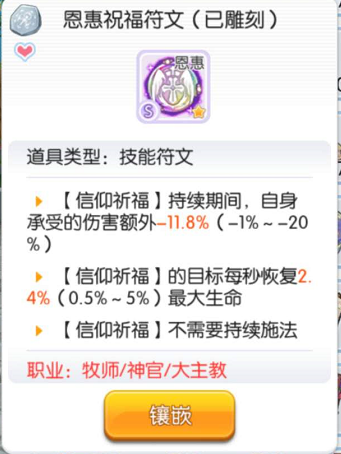顺便给我的师傅上炷香。。。 =​=​=​=​=​=​=​=​=​=​=​=​=​=​=​=​=​=​=​=​=​=​=​=​=​=​=​=​=​=​=​=​=​=​=​=​ 这版本和牧师没什么关系，所以就讲讲符文有关的 =​=​=​=​=​=​=​=​=​=​=​=​=​=​=​=​=​=​=​=​=​=​=​=​=​=​=​=​=​=​=​=​=​=​=​=​ S级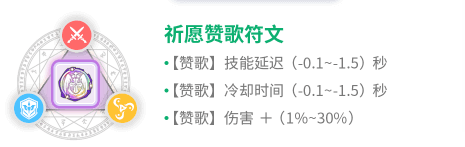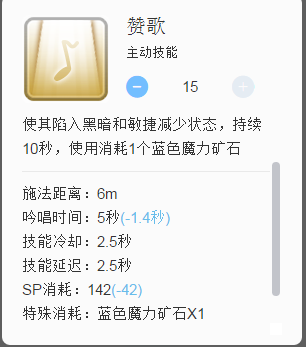祈愿赞歌，不解释，赞歌牧的必备符文，延迟和冷却双低才有用，想要用来挂机可以考虐留一个，说不定下版本加强 美中不足就是一个伤害符文要加个蓝色的小符文就有点说不过去了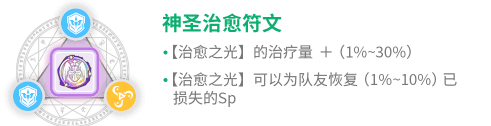神圣治愈，算是一个奶量与功能相结合的符文， 一方面的回蓝提供了66里不能烧热量回蓝的缺点，哪怕是复活起来，只要奶一口就有蓝了，算是弥补了复活术的一个小小缺点，(种树也可以回蓝，有地领呢？？？) 如果单纯只需要奶量，可以只刷第一条，有66需求可以双高，但不是必要暗影克星强行改暗属性，MVP也可以生效，最近的12本老三也特别活跃，肉敏牧改属性，但词条的区间下限挺低的，要刷的加加油，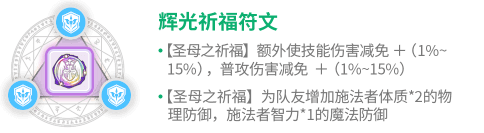辉光祈福别看字多**，就是加技能减免和普攻减免，词条多点防御而已，印象中出来之前被削过，之前是智力*2的魔防，现在只有智力*1， 前两条必须高，有条件的也得要有第三条，(这个词条只是提供一个彩头，不是必须要的)在PVP里比较常用，三个小蓝符文也是挺开心的，可以将防御小符文都往里塞圣光驱毒讲句真真真真话，这符文只有第一个词条能用的，缓毒术因为要读条，持续时间短，驱BUFF技能较多大部分人都没点了， 但这个符文当作是个被动，增加毒抗，第一条词条有多高刷多高，第二第三词条可以无视掉，(个人觉得这个符文PVP需求度较高，不怕毒的职业又多了一个了，可喜可贺)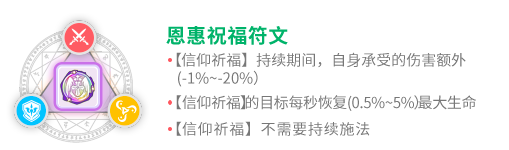恩惠祝福，目前打12本英灵1必带的一个符文，基本没有就相当于一个残废牧师了(不枉我刷了这么久，真香) 必须第三词条 必须第三词条 必须第三词条 第三词条相当于：提 供了可以放其他技能的条件，减少了信仰9秒的CD 第一句话好理解，第二句话如何理解呢？ 以前的信仰需要 不动一直释放9秒的无敌后，才开始读CD 需要靠牺牲才能两个人无中断衔接无敌 如今的信仰， 释放技能后，直接进入CD 不需要牺牲也能两个人无中断衔接无敌 别问了，问就是好用，第1，第2词条可以低，但必须出现第3词条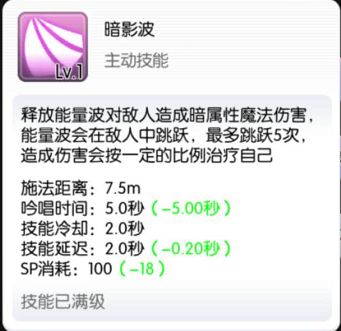光影双生牧师的小**，抢法师的好帮手(头保命)，轮回的好条件 第一词条高，第三词条要有才比较好，像是给牧师提供了一个挂机的技能，但装备要比较好才行，强制暗属性没有点任何增加伤害的方法， 因为基础倍率比较高的缘故，所以也给了轮回号比较好的伤害 优先伤害高的装备，治疗加成增加的伤害并不多(不要硬穿治疗加成的装备)，还是推荐一套的加伤装备比较好， =​=​=​=​=​=​=​=​=​=​=​=​=​=​=​=​=​=​=​=​=​=​=​=​=​=​=​=​=​=​=​=​=​=​=​=​=​=​=​=​=​=​ A级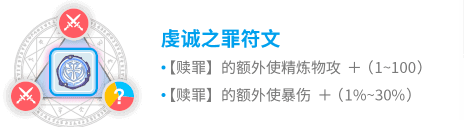虔诚之罪爆牧的第二个加伤符文，能高则高福音赞歌赞歌第二个加伤害的符文，如果想玩赞歌，必须要刷加伤的词条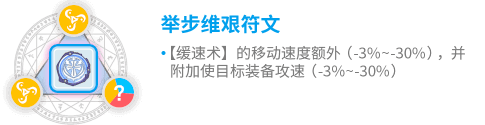举步维艰目前没有格子可以放这个符文，如果碰上了可以缓速的MVP，如12本MVP可以给一下，缓速确实是生效的，配合上火马星效果不错静默祷告暴牧第三个需要的符文，也是PVE必带的符文，增加祷告的的伤害，对暴牧来说就是送了一个祷告LV10，增加的幅度很可观，如遇到高词条的必须要留 =​=​=​=​=​=​=​=​=​=​=​=​=​=​=​=​=​=​=​=​=​=​=​=​=​=​=​=​=​=​=​=​=​=​=​=​=​=​=​=​=​=​=​=​=​=​=​=​ B级驱魔使者，增加驱魔伤害的符文，想玩的可留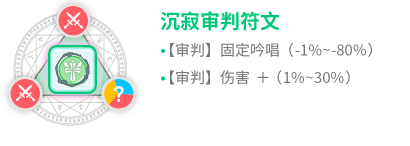沉寂审判，增加伤害和审判效率的符文，想玩可留，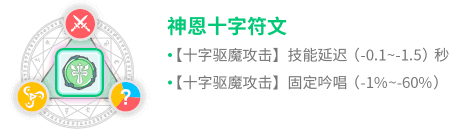神恩十字，配合圣礼与奥服基本接近瞬发，甚至同时有存在超过3个，有意向挂机的可留 =​=​=​=​=​=​=​=​=​=​=​=​=​=​=​=​=​=​=​=​=​=​=​=​=​=​=​=​=​=​=​=​=​=​=​=​=​=​=​=​=​=​=​=​=​=​=​=​ 总体而言，A级符文常用祷告和加穿刺，B级为练级符文。S级鸡肋符文不少，但功能性比较强，像信仰基本上就是改技能 希望大家刷到想刷的符文，命里有时终须有，命里无时莫强求。下个版本见，拜。

 假牧师来暖贴了 等练上去符文也配好就能混234了

 静默祷告恩惠祝福辉光祈福 这是我的目标

 剑骑一真 发表于 2020-2-29 01:13 静默祷告恩惠祝福辉光祈福 这是我的目标 抽到了记得来**

 剑骑一真 发表于 2020-2-29 01:13 静默祷告恩惠祝福辉光祈福 这是我的目标 抽到了记得来还、、愿( ∩'-'⊂ )

 暗影那个，本来是给牧师多一个挂机技能，现在成了全体轮回技能，下水道太惨烈，又抽不到词条高倍率符文，头疼。

 艾妮娅 发表于 2020-2-29 12:24 暗影那个，本来是给牧师多一个挂机技能，现在成了全体轮回技能，下水道太惨烈，又抽不到词条高倍率符文，头 ... 平原3点只挂鼬符文要求不算很高的，800倍+词条肯吃料理+几个核心加伤扭蛋**就足够，收益也比牧师的其他挂法高很多了 就算符文更好无非是去12点，然而12点就算毕业级暗影波也抢不过很多职业（人家一来你就得走），还得错峰挂机（我都被迫弄个地领小号了被炼金恶意抢的没脾气） 其实没必要太在意这个

 才发现那个普攻祷告的第二条有用……

 3394511670发表于 2020-2-29 18:44 才发现那个普攻祷告的第二条有用…… 也就12人英灵用用罢了，其他场景没有非有不可。 然而12人最需要的不是这个，而是6%概率出的信仰第三条符文，没这个英灵不带玩的艾妮娅 发表于 2020-2-29 12:24 暗影那个，本来是给牧师多一个挂机技能，现在成了全体轮回技能，下水道太惨烈，又抽不到词条高倍率符文，头 ... 对的，除非提高小号获得符文的等级，不然真的人均暗影牧

 Flagship雪风发表于 2020-2-29 13:35 平原3点只挂鼬符文要求不算很高的，800倍+词条肯吃料理+几个核心加伤扭蛋**就足够，收益也比牧师的其他挂 ... **的符文，增伤手段少，全靠装备硬件撑上去，但至少能挂一挂机

 Flagship雪风发表于 2020-2-29 18:48 也就12人英灵用用罢了，其他场景没有非有不可。 然而12人最需要的不是这个，而是6%概率出的信仰第三条符 ... 暴牧偶尔用用自动祷告，毕竟是满级的祷告，而且暴牧有用的符文只有三个，信仰是打英灵必备的，没得挑的

 Michiko_Eric 发表于 2020-2-29 21:26 **的符文，增伤手段少，全靠装备硬件撑上去，但至少能挂一挂机 主要增伤全在词条上，词条配13红杖增伤很多。 现在这样能靠比较难获得的13红杖拦住隔壁法师觊觎这个符文的现状还可以，再通过增加增伤手段降低难度的话就成法师快乐符文了。论魔攻装备一般牧师不可能比得过专门玩法系输出的法师贤者

 Flagship雪风发表于 2020-3-1 11:47 主要增伤全在词条上，词条配13红杖增伤很多。 现在这样能靠比较难获得的13红杖拦住隔壁法师觊觎这个符文 ... 所以成为了轮回大户

## 快速回复

 您需要登录后才可以回帖 登录 | 注册 回帖后跳转到最后一页